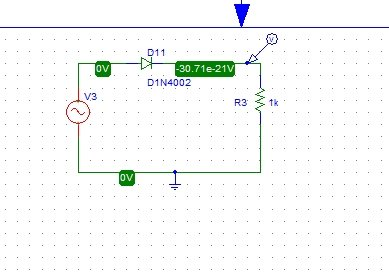# Simulation of rectifiers in Pspice: tutorial 4

In the last tutorial, we have seen how to simulate RL and RC circuits in Pspice. In this tutorial I will help you to simulate a circuit in which a rectifier is designed. Both full wave (bridge) rectifier and half wave rectifiers are designed with the help of PSPICE in this tutorial. At the start of the tutorial a brief introduction regarding rectifiers (both full wave and half wave) is provided. After that he circuits are implemented on PSPICE and the simulation output is verified. Both full wave and half rectifiers are implemented one by one. At the end of the tutorial you are provided with an exercise to do it by yourself, and in the next tutorials I will assume that you have done those exercises and I will not explain the concept regarding them.

### Introduction to Rectifiers

A rectifier is an electrical term and is used to name a circuit which can convert AC (Alternating Current) signal to DC (Direct Current) signal. In simple words a circuit that can convert AC to DC is known as rectifier. The opposite of a rectifier is an inverter. Two main types of a simple rectifier are:

• Half wave rectifier
• Full Wave rectifier

### Half wave rectifier

A half wave rectifier consists of a single diode and a load resistance. It only allows the positive part of an AC signal and during the negative half cycle the diode will act as reverse bias and the voltage at the output resistance will be zero.

### Full wave rectifier

In a full wave rectifier four diodes are connected in the form of a bridge (that’s why called a bridge rectifier) and two of the diodes allow the positive half cycle of the AC signal to pass as it is and the other two diodes changes the direction of the negative half cycle and at the output resistor it also appears as a positive cycle and we get a pulsating DC as you will see shortly in the simulation part.

### Full wave rectifier in PSpice

Figure 1: Opening new schematic

Figure 2: Saving schematic

Figure 3: Getting new part

Figure 4: Diodes

Figure 5: Diode bridge

Figure 6: Input sin

Figure 7: Placing resistor

Figure 8: Ground

Figure 9: Placed components

Figure 10: Complete circuit diagram

Figure 11: Supply attributes

Figure 12: Voltage marker

Figure 13: Voltage marker placed

Figure 14: Analysis setup

Figure 15: Transient properties

Figure 16: Labeling a wire

Figure 17: Simulating the circuit

Figure 18: Output of full wave rectifier

• The output of the full wave rectifier as we have discussed previously is a pulsating DC and not pure DC. Introduction of a capacitor in parallel with the load resistor will result in the conversion of this pulsating DC into pure DC signal. From the graph above it is obvious that the signal at the load resistor is unidirectional i.e. no part of the signal is below the 0 line of the graph (negative), which implies that the rectifier is working properly.
• Lets’ design a half wave rectifier now. A half wave rectifier consists of a single diode connected in series with the input sin voltage and the load resistor, as shown in the figure below,Figure 19: Half wave rectifier

Figure 20: Output of half wave rectifier

• As we have discussed in the introduction part, the negative part of the output of the half wave circuit will be clipped to zero so is obvious from the simulation results.

Exercise:

• Convert the pulsating DC at the output of a full wave bridge rectifier into pure non pulsating DC

(Hint: You can do this with the help of capacitor installation in the circuit)

<<Previous tutorial                                             Next tutorial>>

### 1 thought on “Simulation of rectifiers in Pspice: tutorial 4”

1. 1.How to Simulate 3 phase 3 wire Power system for fault analysis like neutral missing voltage unbalance etc. using Pspice.
2. How to Simulate 3phase star-delta motors starters using Pspice.